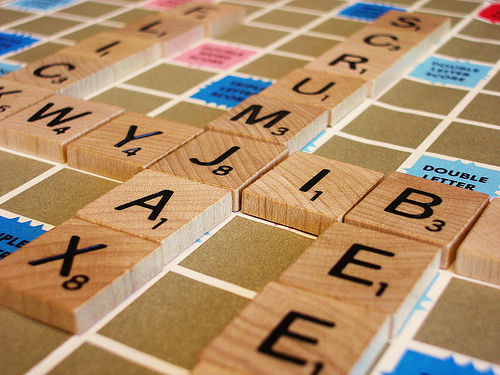Want to share your content on R-bloggers? click here if you have a blog, or here if you don't.

This blog post will do a quick exploration of the grapheme make up of words in the English. Specifically we will use R and the qdap package to answer 3 questions:

1. What is the distribution of word lengths (number of graphemes)?
2. What is the frequency of letter (grapheme) use in English words?
3. What is the distribution of letters positioned within words?

Click HERE for a script with all of the code for this post.We will begin by loading the necessary packages and data (note you will need qdap 2.2.0 or higher):

```if (!packageVersion("qdap") >= "2.2.0") {
install.packages("qdap")
}
library(qdap); library(qdapDictionaries); library(ggplot2); library(dplyr)
```

We will be using `qdapDictionaries::GradyAugmented` to conduct the mini-analysis. The `GradyAugmented` list is an augmented version of Grady Ward’s English words with additions from other various sources including Mark Kantrowitz’s names list. The result is a character vector of 122,806 English words and proper nouns.

```GradyAugmented
```

# Question 1

## What is the distribution of word lengths (number of graphemes)?

To answer this we will use base R’s `summary`, qdap‘s `dist_tab` function, and a `ggplot2` histogram.

```summary(nchar(GradyAugmented))
```
```   Min. 1st Qu.  Median    Mean 3rd Qu.    Max.
1.00    6.00    8.00    7.87    9.00   21.00
```
```dist_tab(nchar(GradyAugmented))
```
```   interval  freq cum.freq percent cum.percent
1         1    26       26    0.02        0.02
2         2   116      142    0.09        0.12
3         3  1085     1227    0.88        1.00
4         4  4371     5598    3.56        4.56
5         5  9830    15428    8.00       12.56
6         6 16246    31674   13.23       25.79
7         7 23198    54872   18.89       44.68
8         8 27328    82200   22.25       66.93
9         9 17662    99862   14.38       81.32
10       10  9777   109639    7.96       89.28
11       11  5640   115279    4.59       93.87
12       12  3348   118627    2.73       96.60
13       13  2052   120679    1.67       98.27
14       14  1066   121745    0.87       99.14
15       15   582   122327    0.47       99.61
16       16   268   122595    0.22       99.83
17       17   136   122731    0.11       99.94
18       18    50   122781    0.04       99.98
19       19    17   122798    0.01       99.99
20       20     5   122803    0.00      100.00
21       21     3   122806    0.00      100.00
```
```ggplot(data.frame(nletters = nchar(GradyAugmented)), aes(x=nletters)) +
geom_histogram(binwidth=1, colour="grey70", fill="grey60") +
theme_minimal() +
colour="blue", alpha=.7) +
xlab("Number of Letters")
```Here we can see that the average word length is 7.87 letters long with a minimum of 1 (expected) and a maximum of 21 letters. The histogram indicates the distribution is skewed slightly right.

# Question 2

## What is the frequency of letter (grapheme) use in English words?

Now we will view the overall letter uses in the augmented Grady Word list. Wheel of Fortune lovers…how will r,s,t,l,n,e fare? Here we will double loop through each word with each letter of the alphabet and grab the position of the letters in the words using `gregexpr`. `gregexpr` is a nifty function that tells the starting locations of regular expressions. At this point the positioning isn’t necessary for answering the 2nd question but we’re setting our selves up to answer the 3rd question. We’ll then use a frequency table and ordered bar chart to see the frequency of letters in the word list.

Be patient with the double loop (`lapply`/`sappy`), it is 122,806 words and takes ~1 minute to run.

```position <- lapply(GradyAugmented, function(x){

z <- unlist(sapply(letters, function(y){
gregexpr(y, x, fixed = TRUE)
}))
z <- z[z != -1]
setNames(z, gsub("\d", "", names(z)))
})

position2 <- unlist(position)

freqdat <- dist_tab(names(position2))
freqdat[["Letter"]] <- factor(toupper(freqdat[["interval"]]),
levels=toupper((freqdat %>% arrange(freq))[] %>% as.character))

ggplot(freqdat, aes(Letter, weight=percent)) +
geom_bar() + coord_flip() +
scale_y_continuous(breaks=seq(0, 12, 2), label=function(x) paste0(x, "%"),
expand = c(0,0), limits = c(0,12)) +
theme_minimal()
```The output is given in percent of letter uses. Let’s see if that jives with the points one gets in a Scrabble game for various tiles:Overall, yeah I suppose the Scrabble point system makes sense. However, it makes me question why the “K” is worth 5 and why “Y” is only worth 3. I’m sure more thought went into the creation of Scrabble than this simple analysis**.

**EDIT: I came across THIS BLOG POST indicating that perhaps the point values of Scrabble tiles are antiquated.

# Question 3

## What is the distribution of letters positioned within words?

Now we will use a heat map to tackle the question of what letters are found in what positions. I like the blue – high/yellow – low configuration of heat maps. For me it is a good contrast but you may not agree. Please switch the high/low colors if they don’t suit.

```dat <- data.frame(letter=toupper(names(position2)), position=unname(position2))

dat2 <- table(dat)
dat3 <- t(round(apply(dat2, 1, function(x) x/sum(x)), digits=3) * 100)
qheat(apply(dat2, 1, function(x) x/length(position2)), high="blue",
low="yellow", by.column=NULL, values=TRUE, digits=3, plot=FALSE) +
ylab("Letter") + xlab("Position") +
guides(fill=guide_legend(title="Proportion"))
```The letters “S” and “C” dominate the first position. Interestingly, vowels and the consonants “R” and “N” lead the second spot. I’m guessing the latter is due to consonant blends. The letter “S” likes most spots except the second spot. This appears to be similar, though less pronounced, for other popular consonants. The letter “R”, if this were a baseball team, would be the utility player, able to do well in multiple positions. One last noticing…don’t put “H” in the third position.

*Created using the reports package